## Thursday, July 30, 2015

### Electromagnetic Fields and Waves by Paul Lorrain, Dale R. Corson and Francois Lorrain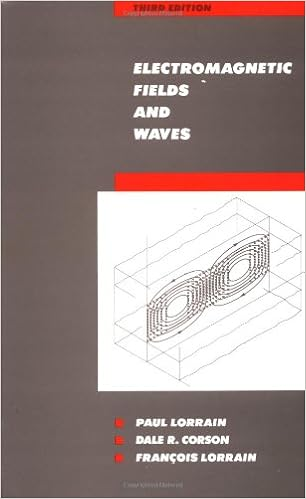Since its original publication in 1962, Lorrain and Corson's text has offered physics and engineering students a formula for developing a working knowledge of the basic principles of electromagnetism. The formula is practice.

### Introduction to Linear Algebra, Fourth Edition by Gilbert Strang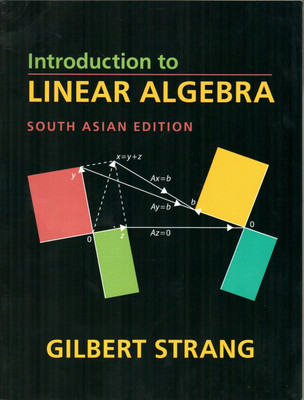Gilbert Strang's textbooks have changed the entire approach to learning linear algebra -- away from abstract vector spaces to specific examples of the four fundamental subspaces: the column space and nullspace of A and A'.
Introduction to Linear Algebra, Fourth Edition includes challenge problems to complement the review problems that have been highly praised in previous editions. The basic course is followed by seven applications: differential equations, engineering, graph theory, statistics, fourier methods and the FFT, linear programming, and computer graphics.

Thousands of teachers in colleges and universities and now high schools are using this book, which truly explains this crucial subject.

Chapter 1: Introduction to Vectors; Chapter 2: Solving Linear Equations; Chapter 3: Vector Spaces and Subspaces; Chapter 4: Orthogonality; Chapter 5: Determinants; Chapter 6: Eigenvalues and Eigenvectors; Chapter 7: Linear Transformations; Chapter 8: Applications; Chapter 9: Numerical Linear Algebra; Chapter 10: Complex Vectors and Matrices; Solutions to Selected Exercises; Final Exam. Matrix Factorizations. Conceptual Questions for Review. Glossary: A Dictionary for Linear Algebra Index Teaching Codes Linear Algebra in a Nutshell.

### Wavelet Transforms: Introduction to Theory & Applications by Raghuveer M. Rao, Ajit S. Bopardikar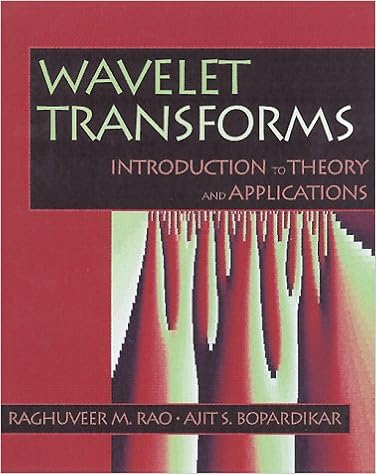*Written by researchers Raghuveer M. Rao and Ajit S. Bopardikar, Wavelet Transforms provides engineers, scientists, and students with a practical understanding of wavelet transforms and their properties. The authors introduce the underlying theory of the transform by presenting a wide range of applications, such as signal processing, image processing, and communications. *This book identifies problems for which wavelet transform techniques are well-suited, shows how to implement wavelet transforms efficiently, and explains how to choose or design appropriate wavelets for a given application. In Chapter 1, basic linear filtering principles are utilized to introduce the reader to continuous wavelet transform. In Chapter 2, the basics of discrete wavelet transforms and multiresolution analysis are presented. Multiresolution analysis is then further explored in Chapter 3. Chapter 4 contains alternative wavelet representations, such as biorthogonal bases, wavelet packets, and multiresolution analysis of images. Chapter 5 provides a detailed treatment of the use of wavelet transform techniques in signal and image compression.In Chapter 6, applications to areas such as denoising, object isolation, and detection are presented. Chapter 7 addresses several more advanced topics, including: choice or design of wavelets for a given application, projection relations for the continuous wavelet transform, biorthogonal bandlimited wavelets, matched wavelet construction, self-similar signals, and linear scale-invariant systems. The supporting disk contains MATLAB routines that enable the reader to experiment with various algorithms and techniques presented in the book. *Practical in their approach, Rao and Bopardikar present the material in a visual and comprehensive manner, using geometric analogies and filtering concepts. The book is written in a language familiar to readers with a basic undergraduate engineering degree.

### Elementary Applied Partial Differential Equations: With Fourier Series and Boundary Value Problems by Richard Haberman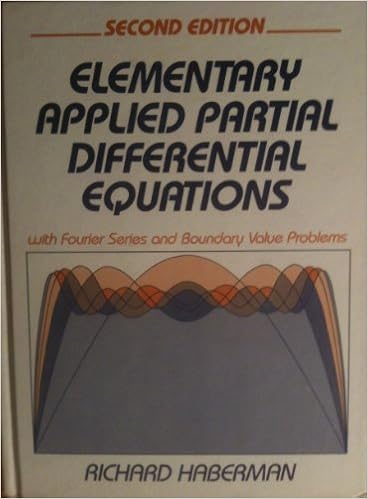This text is designed for engineers, scientists, and mathematicians with a background in elementary ordinary differential equations and calculus.

### A Textbook of Engineering Mathematics: I by H.S. Gangwar and Dr. Prabhakar GuptaTechnical University, Lucknow. All the topics such as Differential equations, Series solution and Special functions, Laplace transform fourier series, Partial differential equations and Applications of partial differential equations are covered in detail. Each unit contains numerous worked out examples along with number of exercise problems. Objectives, Fill in the blanks and matching the answers problems are also provided at end of each exercise. This book is developed as per the latest syllabus of U.P.T.U.

### Methods of Mathematical Physics, Volume 1 by Richard Courant, D. HilbertSince the first volume of this work came out in Germany in 1924, this book, together with its second volume, has remained standard in the field. Courant and Hilbert's treatment restores the historically deep connections between physical intuition and mathematical development, providing the reader with a unified approach to mathematical physics. The present volume represents Richard Courant's second and final revision of 1953.

### Mathematical Methods for Physicists by George Brown Arfken, Hans-Jurgen WeberThis new and completely revised Fourth Edition provides thorough coverage of the important mathematics needed for upper-division and graduate study in physics and engineering. Following more than 28 years of successful class-testing, Mathematical Methods for Physicists is considered the standard text on the subject.
A new chapter on nonlinear methods and chaos is included, as are revisions of the differential equations and complex variables chapters. The entire book has been made even more accessible, with special attention given to clarity, completeness, and physical motivation. It is an excellent reference apart from its course use.

This revised Fourth Edition includes:
Modernized terminology
Group theoretic methods brought together and expanded in a new chapter
An entirely new chapter on nonlinear mathematical physics
Significant revisions of the differential equations and complex variables chapters
Many new or improved exercises
Forty new or improved figures
An update of computational techniques for today's contemporary tools, such as microcomputers, Numerical Recipes, and Mathematica(r), among others

## Wednesday, July 29, 2015

### Special Functions (Encyclopedia of Mathematics and its Applications) by George E. Andrews, Richard Askey, Ranjan Roy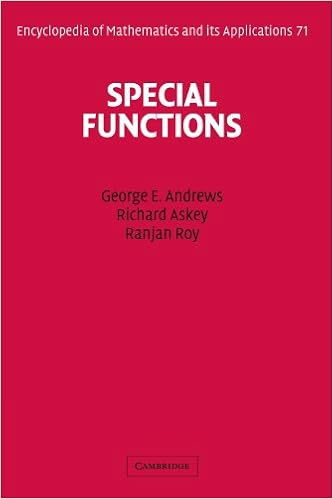Special functions, which include the trigonometric functions, have been used for centuries. Their role in the solution of differential equations was exploited by Newton and Leibniz, and the subject of special functions has been in continuous development ever since. In just the past thirty years several new special functions and applications have been discovered. This treatise presents an overview of the area of special functions, focusing primarily on the hypergeometric functions and the associated hypergeometric series. It includes both important historical results and recent developments and shows how these arise from several areas of mathematics and mathematical physics. Particular emphasis is placed on formulas that can be used in computation. The book begins with a thorough treatment of the gamma and beta functions that are essential to understanding hypergeometric functions. Later chapters discuss Bessel functions, orthogonal polynomials and transformations, the Selberg integral and its applications, spherical harmonics, q-series, partitions, and Bailey chains. This clear, authoritative work will be a lasting reference for students and researchers in number theory, algebra, combinatorics, differential equations, applied mathematics, mathematical computing, and mathematical physics.

### Mathematical Methods for Physics and Engineering Second Edition by K. F. Riley and M. P. Hobson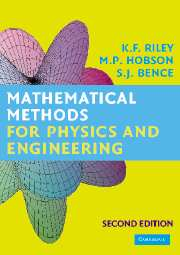The new edition of this highly acclaimed textbook contains several major additions, including more than four hundred new exercises (with hints and answers). To match the mathematical preparation of current senior college and university entrants, the authors have included a preliminary chapter covering areas such as polynomial equations, trigonometric identities, coordinate geometry, partial fractions, binomial expansions, induction, and the proof of necessary and sufficient conditions. Elsewhere, matrix decompositions, nearly-singular matrices and non-square sets of linear equations are treated in detail. The presentation of probability has been reorganized and greatly extended, and includes all physically important distributions. New topics covered in a separate statistics chapter include estimator efficiency, distributions of samples, t- and F- tests for comparing means and variances, applications of the chi-squared distribution, and maximum likelihood and least-squares fitting. In other chapters the following topics have been added: linear recurrence relations, curvature, envelopes, curve-sketching, and more refined numerical methods.

### Mathematical Methods for Physics and Engineering Third Edition by K. F. Riley and M. P. Hobson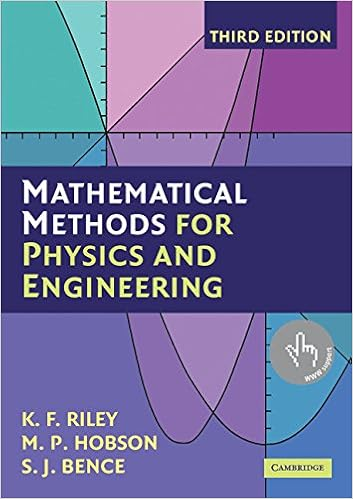Mathematical Methods for Physics and Engineering, Third Edition is a highly acclaimed undergraduate textbook that teaches all the mathematics for an undergraduate course in any of the physical sciences. As well as lucid descriptions of all the topics and many worked examples, it contains over 800 exercises. New stand-alone chapters give a systematic account of the 'special functions' of physical science, cover an extended range of practical applications of complex variables, and give an introduction to quantum operators. This solutions manual accompanies the third edition of Mathematical Methods for Physics and Engineering. It contains complete worked solutions to over 400 exercises in the main textbook, the odd-numbered exercises, that are provided with hints and answers. The even-numbered exercises have no hints, answers or worked solutions and are intended for unaided homework problems; full solutions are available to instructors on a password-protected web site, www.cambridge.org/9780521679718.

### Schaum's Outline of Set Theory and Related Topics Book by Seymour LipschutzConfusing Textbooks? Missed Lectures? Not Enough Time?

Fortunately for you, there's Schaum's Outlines. More than 40 million students have trusted Schaum's to help them succeed in the classroom and on exams. Schaum's is the key to faster learning and higher grades in every subject. Each Outline presents all the essential course information in an easy-to-follow, topic-by-topic format. You also get hundreds of examples, solved problems, and practice exercises to test your skills.

This Schaum's Outline gives you

Practice problems with full explanations that reinforce knowledge
Coverage of the most up-to-date developments in your course field
In-depth review of practices and applications
Fully compatible with your classroom text, Schaum's highlights all the important facts you need to know. Use Schaum's to shorten your study time-and get your best test scores!

Schaum's Outlines-Problem Solved.

### Engineering Mathematics: 5th Edition Book by Dexter Booth and Ken Stroud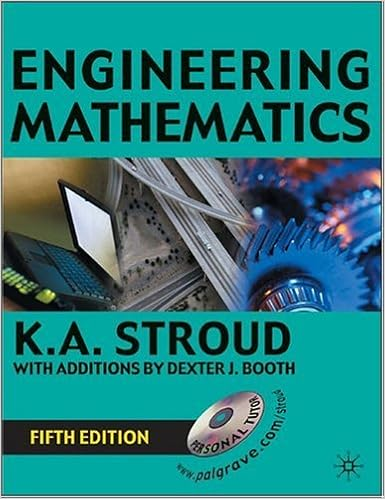Revised to meet the needs of the wide range of students beginning engineering courses, the fifth edition of this text has an extended "Foundation" section including new chapters on graphs, trigonometry, and binomial series and functions. As an introductory mathematics course for students on science and engineering degrees and pre-degree courses, it is suitable for both classroom use and self study. The new CD-ROM included with the book provides stepped hints, worked solutions and immediate feedback on exercises and problems. It has a user friendly interface and intuitive design. A networkable version is also available with a channelling of student progress through to the tutor.

### Advanced Engineering Mathematics by Peter O’Neil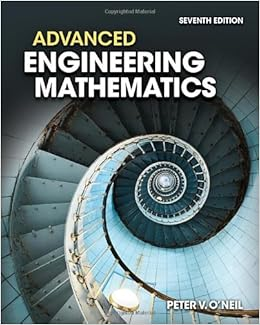Through previous editions, Peter O’Neil has made rigorous engineering mathematics topics accessible to thousands of students by emphasizing visuals, numerous examples, and interesting mathematical models. Now, ADVANCED ENGINEERING MATHEMATICS features revised examples and problems as well as newly added content that has been fine-tuned throughout to improve the clear flow of ideas. The computer plays a more prominent role than ever in generating computer graphics used to display concepts and problem sets. In this new edition, computational assistance in the form of a self contained Maple Primer has been included to encourage students to make use of such computational tools. The content has been reorganized into six parts and covers a wide spectrum of topics including Ordinary Differential Equations, Vectors and Linear Algebra, Systems of Differential Equations and Qualitative Methods, Vector Analysis, Fourier Analysis, Orthogonal Expansions, and Wavelets, and much more.

### Higher Engineering Mathematics by John Bird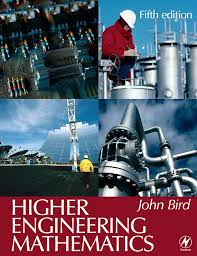John Bird’s approach, based on numerous worked examples and interactive problems, is ideal for students from a wide range of academic backgrounds, and can be worked through at the student’s own pace. Basic mathematical theories are explained in a straightforward manner, being supported by practical engineering examples and applications in order to ensure that readers can relate theory to practice. The extensive and thorough topic coverage makes this an ideal text for a range of university degree modules, foundation degrees, and HNC/D units.

Now in its sixth edition, Higher Engineering Mathematics is an established textbook that has helped many thousands of students to gain exam success. It has been updated to maximise the book’s suitability for first year engineering degree students and those following foundation degrees. This book also caters specifically for the engineering mathematics units of the Higher National Engineering schemes from Edexcel. As such it includes the core unit, Analytical Methods for Engineers, and two specialist units, Further Analytical Methods for Engineers and Engineering Mathematics, both of which are common to the electrical/electronic engineering and mechanical engineering pathways. For ease of reference a mapping grid is included that shows precisely which topics are required for the learning outcomes of each unit.

### Handbook of Mathematics for Engineers and Scientists. ByAndrei D. Polyanin and Alexan der V. Manzhirov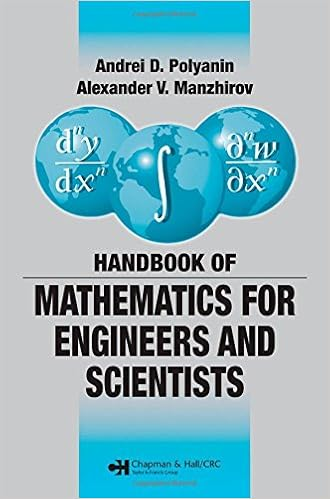The Handbook of Mathematics for Engineers and Scientists covers the main fields of mathematics and focuses on the methods used for obtaining solutions of various classes of mathematical equations that underlie the mathematical modeling of numerous phenomena and processes in science and technology. To accommodate different mathematical backgrounds, the preeminent authors outline the material in a simplified, schematic manner, avoiding special terminology wherever possible.
Organized in ascending order of complexity, the material is divided into two parts. The first part is a coherent survey of the most important definitions, formulas, equations, methods, and theorems. It covers arithmetic, elementary and analytic geometry, algebra, differential and integral calculus, special functions, calculus of variations, and probability theory. Numerous specific examples clarify the methods for solving problems and equations. The second part provides many in-depth mathematical tables, including those of exact solutions of various types of equations.

This concise, comprehensive compendium of mathematical definitions, formulas, and theorems provides the foundation for exploring scientific and technological phenomena.

### Advanced Engineering Mathematics Book by Dennis G. ZillNow with a full-color design, the new Fourth Edition of Zill's Advanced Engineering Mathematics provides an in-depth overview of the many mathematical topics necessary for students planning a career in engineering or the sciences. A key strength of this text is Zill's emphasis on differential equations as mathematical models, discussing the constructs and pitfalls of each. The Fourth Edition is comprehensive, yet flexible, to meet the unique needs of various course offerings ranging from ordinary differential equations to vector calculus. Numerous new projects contributed by esteemed mathematicians have been added. New modern applications and engaging projects makes Zill's classic text a must-have text and resource for Engineering Math students!

### Advanced engineering mathematics Book by Ken Stroud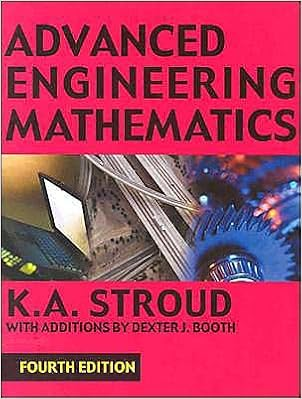This revised and expanded best-selling advanced engineering mathematics textbook offers everything a student needs. Including four new topics and with several other topics extended in their coverage, this is a comprehensive course for all undergraduates in engineering and science from second year level onwards. Its highly successful technique-oriented approach guides the student through the development of each topic. There are hundreds of worked examples and exercises. Advanced Engineering Mathematics is the new edition of Further Engineering Mathematics (third edition), and companion volume to Engineering Mathematics (fifth edition) which has sold more than half a million copies world-wide.

## Monday, July 27, 2015

### Advanced Engineering Mathematics Book by Erwin Kreyszig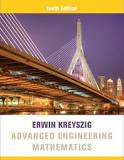A revision of the market leader, Kreyszig is known for its comprehensive coverage, careful and correct mathematics, outstanding exercises, helpful worked examples, and self-contained subject-matter parts for maximum teaching flexibility. The new edition provides invitations - not requirements - to use technology, as well as new conceptual problems, and new projects that focus on writing and working in teams.

### A Course in Mathematics by Woods and BaileyPublished in 1907
Topics include natural sciences, Mathematics, Fundamental and general consideration of mathematics
Publisher : Ginn And Company.
Pages: 409
Language: English
Book contributor: Osmania University

### The Fourth State of Matter: An Introduction to Plasma Science by Shalom Eliezer, Y Eliezer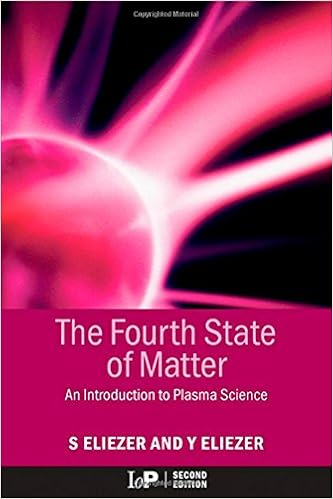Plasma physics may hold the key to a virtually inexhaustible future energy source through the control of thermonuclear reactions. The complexity of plasma physics makes it a difficult subject to write about in popular terms, but the authors of The Fourth State of Matter: An Introduction to Plasma Science, Second Edition treat plasma in a comprehensible way.

Combining factual substance with an easy-to-read and lucid presentation, the book describes the characteristics of plasma, its distribution in the universe, and how it is used by man. It explores plasma in industry and presents current and possible future applications. The book also details the history of plasma research and plasma technology. Retained from the first edition, rhyming verses add fun to the explanation of what can be complicated scientific concepts.

Accessible to anyone interested in plasma physics, this non-mathematical book is an excellent addition to a well-rounded science collection.

### Introduction to solid state physics Book by Charles KittelSince the publication of the first edition over 50 years ago, Introduction to Solid State Physics has been the standard solid state physics text for physics students. The author's goal from the beginning has been to write a book that is accessible to undergraduates and consistently teachable. The emphasis in the book has always been on physics rather than formal mathematics. With each new edition, the author has attempted to add important new developments in the field without sacrificing the book's accessibility and teachability.

A very important chapter on nano-physics has been written by an active worker in the field. This field is the liveliest addition to solid state science during the past ten years

The text uses the simplifications made possible by the wide availability of computer technology. Searches using keywords on a search engine (such as Google) easily generate many fresh and useful references

### Plasma Physics: An Introduction by Richard Fitzpatrick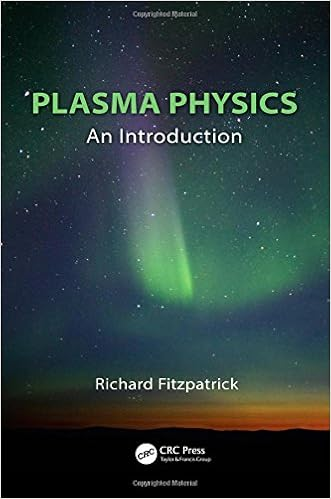Plasma Physics: An Introduction is based on a series of university course lectures by a leading name in the field, and thoroughly covers the physics of the fourth state of matter. This book looks at non-relativistic, fully ionized, non-degenerate, quasi-neutral, and weakly coupled plasma. Intended for the student market, the text provides a concise and cohesive introduction to plasma physics theory, and offers a solid foundation for students wishing to take higher level courses in plasma physics.

### Introduction to Modern Solid State Physics by Yuri M. Galperin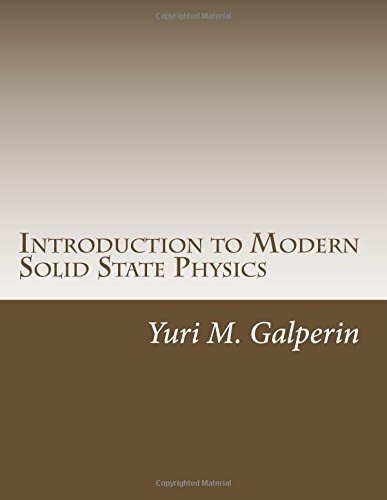This introduction to modern solid state physics covers basic concepts (geometry of lattices, lattice vibrations, electrons in a lattice), normal metals and semiconductors, basics of quantum transport, and superconductivity.

## Sunday, July 26, 2015

### Fundamentals of Plasma Physics Book by Paul Murray Bellan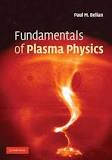This rigorous explanation of plasmas is relevant to diverse plasma applications such as controlled fusion, astrophysical plasmas, solar physics, magnetospheric plasmas, and plasma thrusters. More thorough than previous texts, it exploits new powerful mathematical techniques to develop deeper insights into plasma behavior. After developing the basic plasma equations from first principles, the book explores single particle motion with particular attention to adiabatic invariance. The author then examines types of plasma waves and the issue of Landau damping. Magnetohydrodynamic equilibrium and stability are tackled with emphasis on the topological concepts of magnetic helicity and self-organization. Advanced topics follow, including magnetic reconnection, nonlinear waves, and the Fokker-Planck treatment of collisions. The book concludes by discussing unconventional plasmas such as non-neutral and dusty plasmas. Written for beginning graduate students and advanced undergraduates, this text emphasizes the fundamental principles that apply across many different contexts.

## Friday, July 24, 2015

### The Art of Electronics Book by Paul Horowitz and Winfield Hill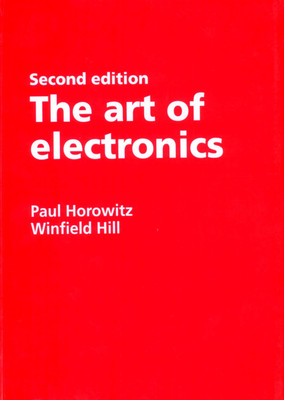The book covers many areas of electronics design, from basic DC voltage, current, and resistance, to active filters and oscillators, to digital electronics, including microprocessors and digital bus interfacing. It also includes discussions of such often-neglected areas as high-frequency, high-speed design techniques and low-power applications.

The book includes many example circuits. In addition to having examples of good circuits, it also has examples of bad ideas, with discussions of what makes the good designs good and the bad ones bad. It can be described as a cross between a textbook and reference manual, though without the chapter-end questions and exercises which are often found in textbooks.

## Thursday, July 23, 2015

### Fundamentals of Geophysics by William Lowrie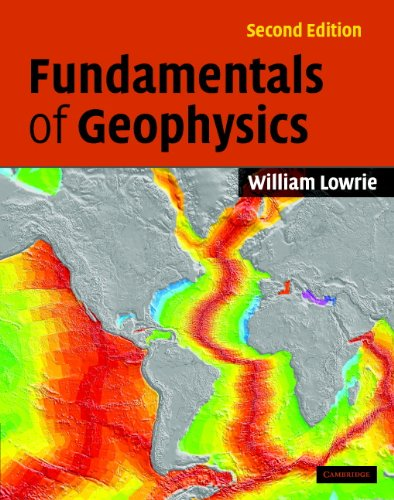This textbook manages to bear the weight of the complex mathematics associated with the study of the Earth's surface and interior. William Lowrie simplifies the math to about second-year degree level making this an excellent textbook. The book is well illustrated and clearly presented. This is the best and most comprehensive general geophysics book available, not only for undergraduate students but also for more experienced graduate students. It is clearly written, beautifully illustrated, and has the appropriate balance between mathematical and descriptive treatment. The new second edition includes text boxes that deal concisely with specific background topics (e.g. Fourier transforms), as well as useful exercises for students at the end of each chapter.

### Classical Mechanics Book by Herbert Goldstein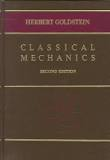Classical Mechanics is a textbook about classical mechanics written by Herbert Goldstein. The scope of the book is undergraduate and graduate level. Since its first publication in 1950, it has been one of the standard references in its subject around the world.

### Introductory Quantum Mechanics Book by Richard LiboffCareful and detailed explanations of challenging concepts in Introductory Quantum Mechanics, Fourth Edition, and comprehensive and up-to-date coverage, continue to set the standard in physics education.

## Wednesday, July 22, 2015

### Physics of Light and OpticsPhysics of Light and Optics is a high-quality free textbook designed for an advanced undergraduate optics course for physics majors. It is used at universities around the world.

### Fundamentals of Optics Book by Francis Jenkins and Harvey Elliott White

1.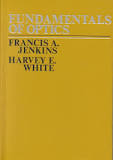2. An introduction to geometrical, physical, and quantum optics

### Fundamentals of Physics Book by David Halliday, Jearl Walker, and Robert Resnick

1.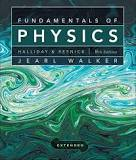2. Fundamentals of Physics is a calculus-based physics textbook by David Halliday, Robert Resnick, and Jearl Walker. The textbook is currently in its tenth edition (published 2013) and is published in a five-volume set. The current version is a revised version of the original textbook Physics by Halliday and Resnick, first published in 1960. It is widely used in colleges as part of the undergraduate physics courses, and has been well known to science and engineering students for decades as "the gold standard" offreshman-level physics texts. In 2002, the American Physical Society named the work the most outstanding introductory physics text of the 20th century.

The most recent editions of the textbook have been enhanced by Walker to include Checkpoint questions. These are conceptual ranking-task questions that help the student before embarking on numerical calculations.

The textbook covers most of the basic topics in physics:

Mechanics

Waves

Thermodynamics
Electromagnetism
Optics
Special Relativity
The extended edition also contains introductions to topics such as quantum mechanics, atomic theory, solid-state physics, nuclear physics and cosmology. A solutions manual and a study guide are also available.